Updating search results...

# 10 Results

View
Selected filters:
• scientific-notation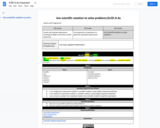Conditional Remix & Share Permitted
CC BY-NC-SA
Rating
0.0 stars

Perform operations with numbers expressed in scientific notation, including problems where both decimal and scientific notation are used.

Subject:
Mathematics
Material Type:
Activity/Lab
Author:
Liberty Public Schools
04/13/2021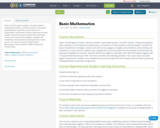Unrestricted Use
CC BY
Rating
0.0 stars

Topics include signed numbers, decimal numbers, exponential notation, scientific notation, solving and graphing linear equations, an introduction to polynomials, and systems of linear equations and their graphs. Geometrical topics include lines and angles, closed curves and convex polygons, triangles and similarities, and symmetry and proportion in nature and art. All course content by Valerie Dietel-Brenneman. Content added to OER Commons by Victoria Vidal.

Subject:
Mathematics
Numbers and Operations
Material Type:
Syllabus
Provider:
Roxbury Community College
05/10/2022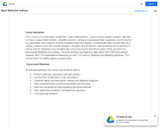Unrestricted Use
CC BY
Rating
0.0 stars

This course is a continuation of MAT087, Basic Mathematics. Topics include signed numbers, decimal numbers, exponential notation, scientific notation, solving and graphing linear equations, an introduction to polynomials, and systems of linear equations and their graphs. Geometrical topics include lines and angles, closed curves and convex polygons, triangles and similarities, and symmetry and proportion in nature and art. Students may complete this course during the first three weeks of the semester by passing the MyMathLab modules. Students will then be eligible to take either MAT 099 Intermediate Algebra, MAT 114-Quantitative Reasoning or MAT 120-Intro to Statistics the following semester. This course does not satisfy degree requirements.

Subject:
Mathematics
Material Type:
Full Course
Provider:
Northern Essex Community College
Author:
Jim Sullivan
05/15/2019Unrestricted Use
CC BY
Rating
0.0 stars

Topics include signed numbers, decimal numbers, exponential notation, scientific notation, solving and graphing linear equations, an introduction to polynomials, and systems of linear equations and their graphs. Geometrical topics include lines and angles, closed curves and convex polygons, triangles and similarities, and symmetry and proportion in nature and art. Students may complete this course during the first three weeks of the semester by passing the MyMathLab modules. Students will then be eligible to take either MAT 099 Intermediate Algebra, MAT 114-Quantitative Reasoning or MAT 120-Intro to Statistics the following semester. This course does not satisfy degree requirements. Students may complete this course during the first three weeks of the semester by passing the MyOpenMath Acceleration assignments.

Subject:
Mathematics
Material Type:
Full Course
Provider:
Roxbury Community College
Author:
Valerie Dietel-Brenneman
05/15/2019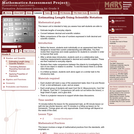Only Sharing Permitted
CC BY-NC-ND
Rating
0.0 stars

This lesson unit is intended to help teachers assess how well students are able to: estimate lengths of everyday objects; convert between decimal and scientific notation; and make comparisons of the size of numbers expressed in both decimal and scientific notation.

Subject:
Mathematics
Material Type:
Assessment
Lesson Plan
Provider:
Shell Center for Mathematical Education
Provider Set:
Mathematics Assessment Project (MAP)
04/26/2013Unrestricted Use
Public Domain
Rating
0.0 stars

This is a booklet containing 37 space science mathematical problems, several of which use authentic science data. The problems involve math skills such as unit conversions, geometry, trigonometry, algebra, graph analysis, vectors, scientific notation, and many others. Learners will use mathematics to explore science topics related to Earth's magnetic field, space weather, the Sun, and other related concepts. This booklet can be found on the Space Math@NASA website.

Subject:
Astronomy
Mathematics
Physical Science
Material Type:
Lesson Plan
Provider:
NASA
Provider Set:
Space Math
11/05/2014Conditional Remix & Share Permitted
CC BY-NC-SA
Rating
0.0 stars

In this lesson, through various examples and activities, exponential growth and polynomial growth are compared to develop an insight about how quickly the number can grow or decay in exponentials. A basic knowledge of scientific notation, plotting graphs and finding intersection of two functions is assumed.

Subject:
Education
Functions
Mathematics
Material Type:
Lecture
Provider:
MIT
Provider Set:
MIT Blossoms
Author:
MIT BLOSSOMS
07/12/2014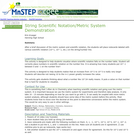Conditional Remix & Share Permitted
CC BY-NC-SA
Rating
0.0 stars

Short demonstration on scientific notation by asking students to place numbers on a number line using string and notecards.

Subject:
Life Science
Physical Science
Material Type:
Activity/Lab
Provider:
Science Education Resource Center (SERC) at Carleton College
Provider Set:
Pedagogy in Action
Author:
Erin Krueger
08/10/2012Conditional Remix & Share Permitted
CC BY-NC-SA
Rating
0.0 stars

A Khan Academy module that offers instruction and practice problems in exponents and scientific notation.

Subject:
Mathematics
Material Type:
Activity/Lab
Interactive
Lecture Notes
Provider:
Provider Set:
Author:
Salman Khan
06/07/2019Conditional Remix & Share Permitted
CC BY-SA
Rating
0.0 stars

A short video that briefly explains the concept of powers of ten and scientific notation using money as an analogy.

Subject:
Astronomy
Physical Science
Material Type:
Lesson
Author: